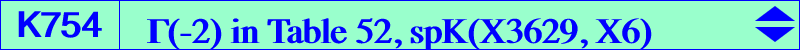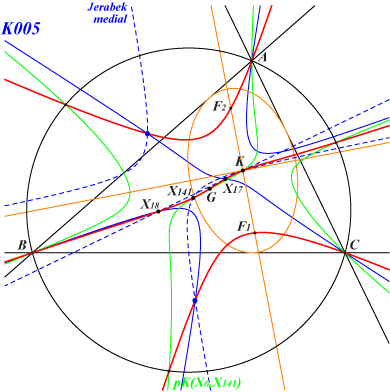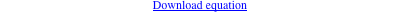∑ (y - z) [a^4 y z + b^2 c^2 x (- 2x + y + z)] = 0X(2), X(6), X(17), X(18), X(141) (blue) isogonal conjugates of the Ix-anticevian points, see table 23 foci of the K-ellipse (inellipse with center K when the triangle ABC is acute angle)The circular quartics Q002 and Q056 have the same four points on the line at infinity hence they must meet again at twelve finite points which are A, B, C, X(6), the four foci of the inconic with center X(6) and the isogonal conjugates of the four Ix-anticevian points. See Table 23. K754 meets the line at infinity and the circumcircle at the same points as pK(X6, X3629) and K655 = pK(X6, X141) respectively. The isogonal transform of K754 is K755. These two cubics belong to the Brocard-Grebe pencil where they are denoted by Γ(-2) and Γ(2) respectively. See Table 52. K754 is spK(X3629, X6) in CL055.